# 详解机器学习中的梯度消失、爆炸原因及其解决方法

## 前言

- 预训练加微调
- 梯度剪切、权重正则（针对梯度爆炸）
- 使用不同的激活函数
- 使用batchnorm
- 使用残差结构
- 使用LSTM网络


# 第一部分：为什么要使用梯度更新规则

$F(x) = {f_n}(...{f_3}({f_2}({f_1}(x)*{\theta _1} + b)*{\theta _2} + b)...)$ 我们最终的目的是希望这个多元函数可以很好的完成输入到输出之间的映射，假设不同的输入，输出的最优解是$g(x)$ ，那么，优化深度网络就是为了寻找到合适的权值，满足$Loss = L(g(x),F(x))$取得极小值点，比如最简单的损失函数
$Loss = ||g(x)-f(x)||^2_2$,假设损失函数的数据空间是下图这样的，我们最优的权值就是为了寻找下图中的最小值点，对于这种数学寻找最小值问题，采用梯度下降的方法再适合不过了。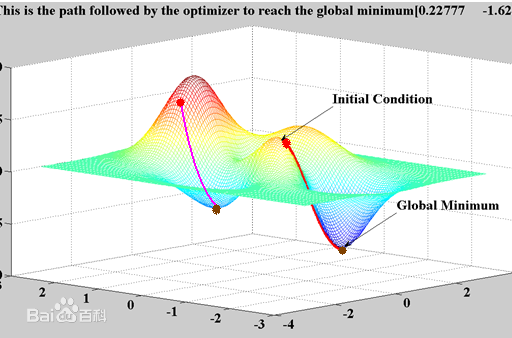# 第二部分：梯度消失、爆炸

### 1.深层网络角度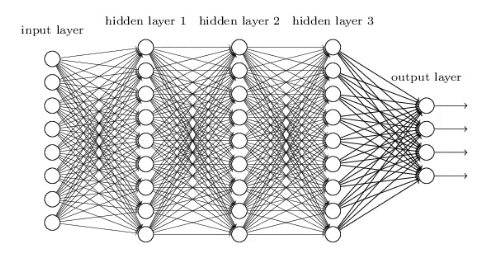BP算法基于梯度下降策略，以目标的负梯度方向对参数进行调整，参数的更新为$w \leftarrow w+\Delta w$，给定学习率$\alpha$，得出$\Delta w=-\alpha \frac{\partial Loss}{\partial w}$。如果要更新第二隐藏层的权值信息，根据链式求导法则，更新梯度信息：
$\Delta w_2=\frac{\partial Loss}{\partial w_2}=\frac{\partial Loss}{\partial f_4}\frac{\partial f_4}{\partial f_3}\frac{\partial f_3}{\partial f_2}\frac{\partial f_2}{\partial w_2}$，很容易看出来$\frac{\partial f_2}{\partial w_2}=\frac{\partial f}{\partial (f_1*w_2)}f_1$，即第二隐藏层的输入。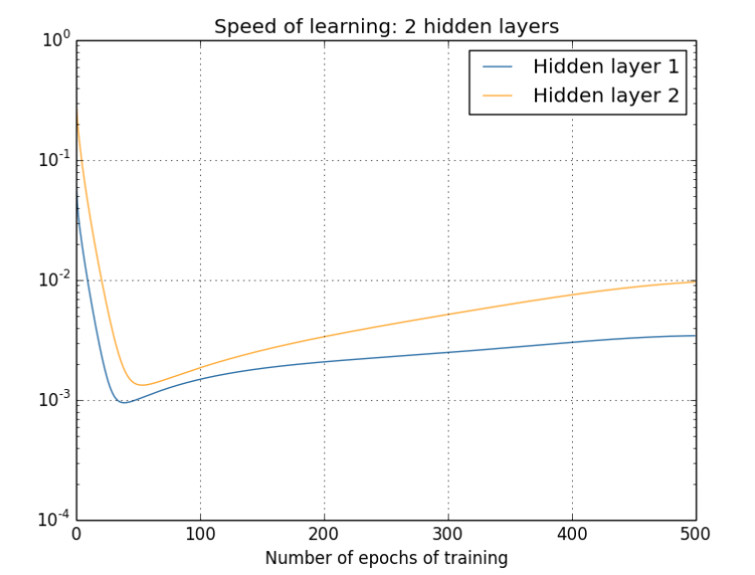那么对于四个隐层的网络来说，就更明显了，第四隐藏层比第一隐藏层的更新速度慢了两个数量级：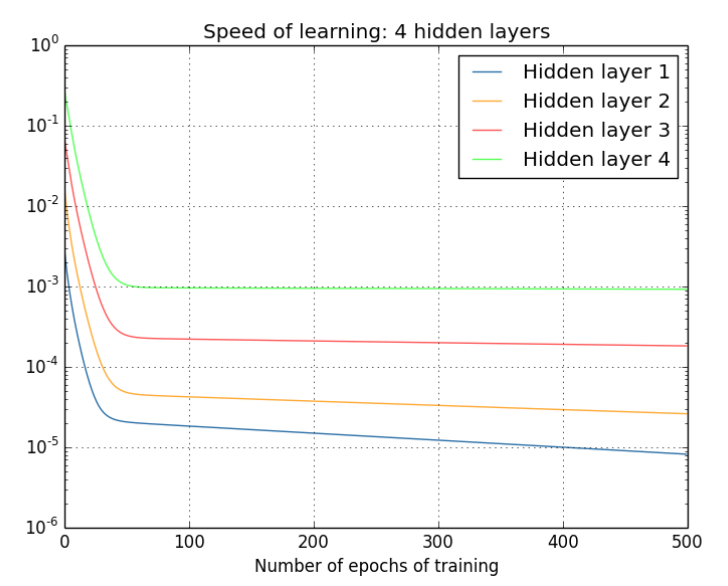### 2.激活函数角度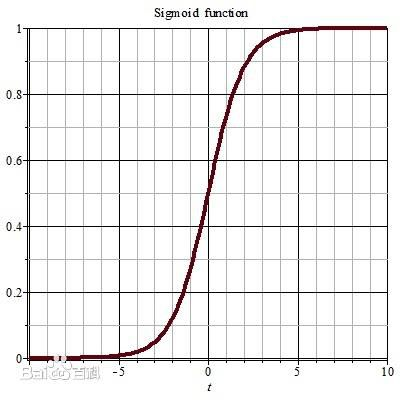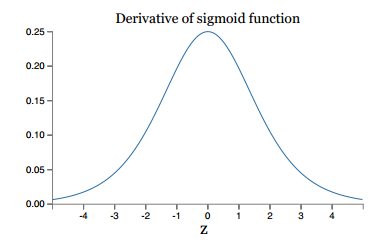同理，tanh作为激活函数，它的导数图如下，可以看出，tanh比sigmoid要好一些，但是它的导数仍然是小于1的。tanh数学表达为：


$tanh(x)=\frac{e^x-e^{-x}}{e^x+e^{-x}}$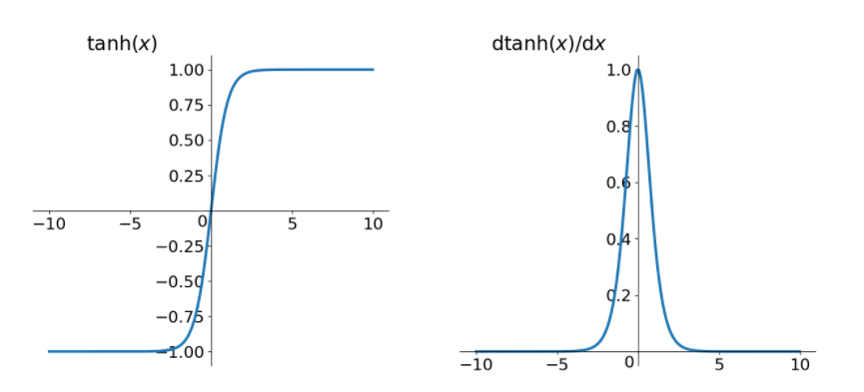# 第三部分：梯度消失、爆炸的解决方案

### 2.2 方案2-梯度剪切、正则

注：在WGAN中也有梯度剪切限制操作，但是和这个是不一样的，WGAN限制梯度更新信息是为了保证lipchitz条件。


regularization_loss = tf.add_n(tf.losses.get_regularization_losses(scope='my_resnet_50'))


    l2_loss = tf.add_n([tf.nn.l2_loss(var) for var in tf.trainable_variables() if 'weights' in var.name])



$Loss=(y-W^Tx)^2+ \alpha ||W||^2$

注：事实上，在深度神经网络中，往往是梯度消失出现的更多一些。


### 2.3 方案3-relu、leakrelu、elu等激活函数

**Relu:**思想也很简单，如果激活函数的导数为1，那么就不存在梯度消失爆炸的问题了，每层的网络都可以得到相同的更新速度，relu就这样应运而生。先看一下relu的数学表达式：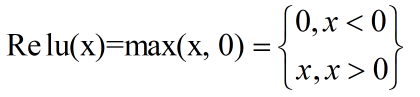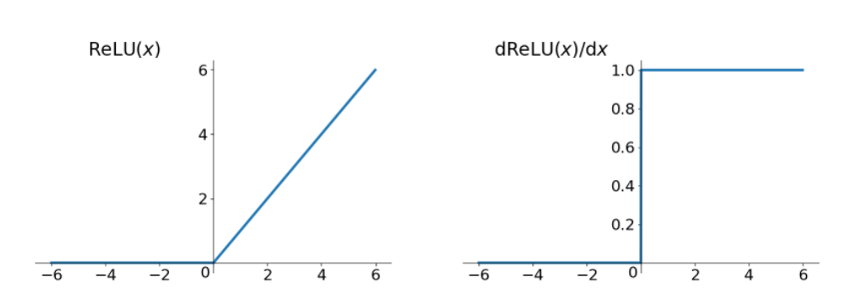relu的主要贡献在于：

 -- 解决了梯度消失、爆炸的问题
-- 计算方便，计算速度快
-- 加速了网络的训练


-- 由于负数部分恒为0，会导致一些神经元无法激活（可通过设置小学习率部分解决）
-- 输出不是以0为中心的


leakrelu
leakrelu就是为了解决relu的0区间带来的影响，其数学表达为：$leakrelu=max(k*x,x)$其中k是leak系数，一般选择0.01或者0.02，或者通过学习而来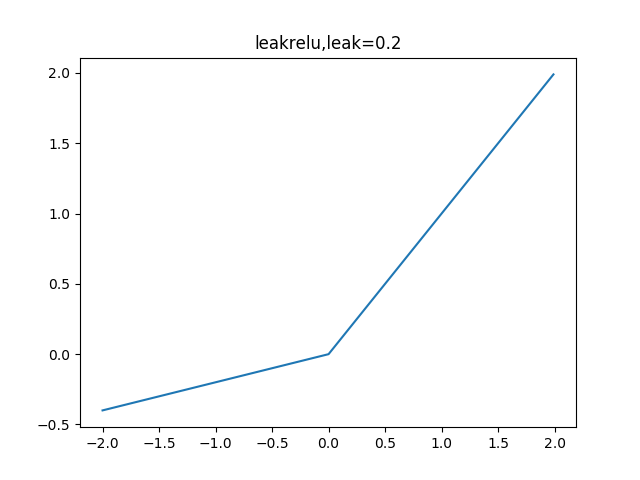leakrelu解决了0区间带来的影响，而且包含了relu的所有优点
elu
elu激活函数也是为了解决relu的0区间带来的影响，其数学表达为：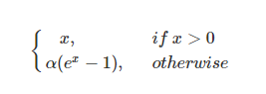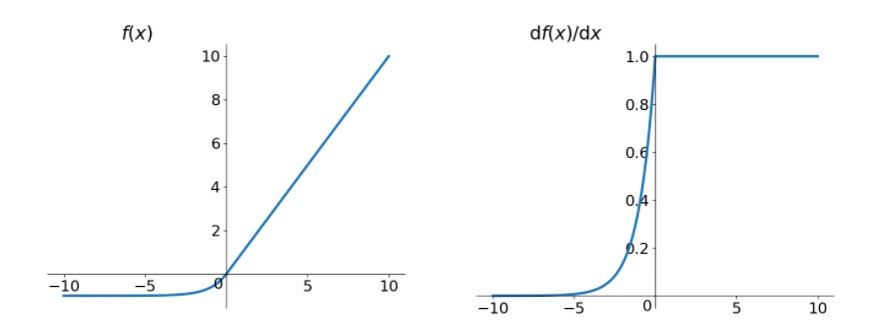### 2.4 解决方案4-batchnorm

Batchnorm是深度学习发展以来提出的最重要的成果之一了，目前已经被广泛的应用到了各大网络中，具有加速网络收敛速度，提升训练稳定性的效果，Batchnorm本质上是解决反向传播过程中的梯度问题。batchnorm全名是batch normalization，简称BN，即批规范化，通过规范化操作将输出信号x规范化保证网络的稳定性。

http://blog.csdn.net/qq_25737169/article/details/79048516

### 2.5 解决方案5-残差结构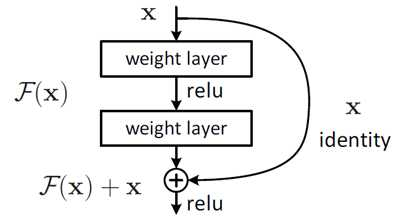注：上面的推导并不是严格的证明。


### 2.6 解决方案6-LSTM

LSTM全称是长短期记忆网络（long-short term memory networks），是不那么容易发生梯度消失的，主要原因在于LSTM内部复杂的“门”(gates)，如下图，LSTM通过它内部的“门”可以接下来更新的时候“记住”前几次训练的”残留记忆“，因此，经常用于生成文本中。目前也有基于CNN的LSTM，感兴趣的可以尝试一下。## 参考资料:

1.《Neural networks and deep learning》
2.《机器学习》周志华
3. https://www.cnblogs.com/willnote/p/6912798.html
4. https://www.zhihu.com/question/38102762
5. http://www.jianshu.com/p/9dc9f41f0b29